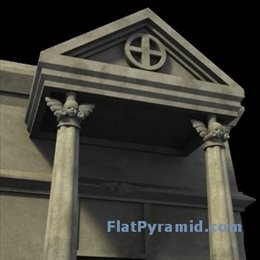# Types Of 3D Modeling: Polygonal Modeling

There are three common shapes created with the use of polygonal 3D modeling; triangles, quads, and elements. Triangles are formed when three sided polygons are uses, quads are formed with four sided polygons are used, and an element is created by a group of polygons connected together at a shared point.
Polygon modeling refers to 3D modeling which use polygons to create the shell of a 3D model. The polygons are used to create the mesh surface with the uses of vertexes in a linear pattern.

>Mesh 3D modeling uses vertexes as coordination points on the surface where three of the five surfaces of the polygon are attached to one another. Two of these vertexes that become an edge are connected by a straight line and then, each one of the polygons that are used to create the 3D modeling figure.

Polygons are the most adept form of 3D modeling for a computer to create. They can be textured or create the appearance of curved surfaces with the use of many tiny lines.
Primitives are the shapes formed within the program within the modeling environment that can be used to create a mesh.

3D modeling primitives consist of spheres, cylinders, cubes, squares, triangles and discs. Spheres are created with the use of multiple triangles to create the curved surface required for the round representation.

There are six basic operations formed in polygonal modeling. Creations refer to a new geometrical shape being formed from another mathematical object. Lofting refers to the action of generating a mesh by continuing a shape over a pattern. Extruding also copies a shape, but over the period of a line rather than a space.

Revolving refers to using a shape to rotating and copying the shape around a specific point and lastly, marching cubes which can create shapes using specific algorithms.